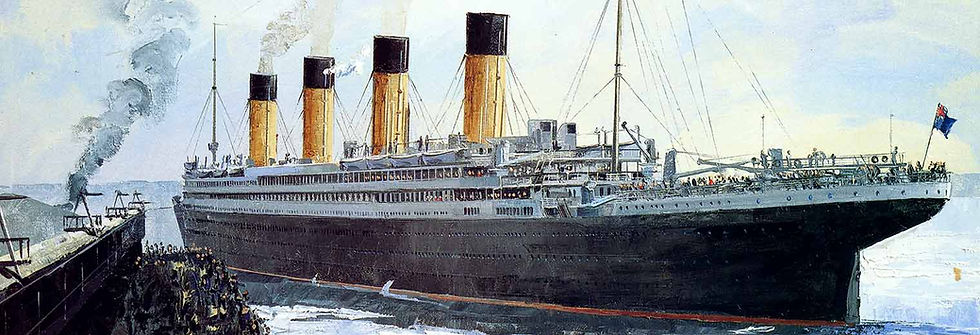top of page
Search

# Titanic Survival Dataset - Classification

Updated: Nov 1, 2021## Description :

This dataset provides information on the fate of passengers on the Titanic, summarized according to economic status (class), sex, age and survival. This dataset can be used for classifying passengers into two groups based on whether they survived or not.

## Recommended Model :

Algorithms to be used: random forest, svm, logistic regression etc

Recommended Project :

Titanic survival prediction

https://www.kaggle.com/c/titanic/data

## Overview of data

Detailed overview of dataset:

- Rows = 1309 ( train set: 891, test set: 418)

- Columns= 1 2

1. PassengerId: Passenger identification number

2. Survival : Passenger's survival (0= No; 1 = Yes)

3. Pclass: Passenger's Class (1 = 1st; 2 = 2nd; 3 = 3rd)

4. name: Passenger's Name

5. sex: Passenger's Sex

6. age: Passenger's Age

7. sibsp: Number of Siblings/Spouses Aboard

8. parch: Number of Parents/Children Aboard

9. ticket: Ticket Number

10. fare: Passenger's Fare (British pound)

11. cabin: Cabin

12. embarked: Port of Embarkation (C = Cherbourg; Q = Queenstown; S = Southampton)

NOTE:

pclass: A proxy for socio-economic status (SES):

1st = Upper

2nd = Middle

3rd = Lower

age: Age is fractional if less than 1. If the age is estimated, it is in the form of xx.5

sibsp: The dataset defines family relations in this way:

Sibling = brother, sister, stepbrother, stepsister

Spouse = husband, wife (mistresses and fiancés were ignored)

parch: The dataset defines family relations in this way:

Parent = mother, father

Child = daughter, son, stepdaughter, stepson

Some children travelled only with a nanny, therefore parch=0 for them.

## EDA [CODE]

```import pandas as pd```# check details of the dataframe
data.info()``````# check the no. of missing values in each column
data.isna().sum()``````# statistical information about the dataset
data.describe()``````# data distribution

import seaborn as sns
import matplotlib.pyplot as plt

sns.countplot(x='Survived', data=data)
plt.show()

sns.countplot(x='Pclass', hue='Survived', data=data)
plt.show()

sns.countplot(x='Sex', hue='Survived', data=data)
plt.show()

fig = sns.FacetGrid(data, col='Survived', hue='Survived', height=4)
fig.map(sns.histplot, 'Age', bins=30, kde=False)
plt.show()

fig = sns.FacetGrid(data, col='Survived', hue='Survived', height=4)
fig.map(sns.histplot, 'Fare', bins=30, kde=False)
plt.show()

sns.countplot(x='SibSp',hue='Survived', data=data)
plt.show()

sns.countplot(x='Parch',hue='Survived', data=data)
plt.legend(title='Survived' ,loc='upper right')
plt.show()

sns.countplot(x='Embarked', hue='Survived', data=data)
plt.show()

```

## Other datasets for classification:

If you need implementation for any of the topics mentioned above or assignment help on any of its variants, feel free to contact us.Tags:

bottom of page